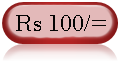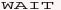Web Development : Starting from Concept, HTML, CSS, Web Site Develpment to Website Launching.
Written by: Sanjay Srivastava
B.Tech (Honours);IIT Kharagpur
PMP ; PMI USA

Class - VIII - Maths:- INDEX

1. Squares & Square Roots
2. Cubes & Cube Roots
4. Profit Loss & Discount
5. Compound Interest
6. Algebraic Identities7. Polynomials8. Linear Equations9. Parallel Lines10. Types of Quadrilaterals11. Construction of Quadrilaterals12. Circles13. Areas14. Surface Areas15. Volumes16. StatisticsClass - VIII - Maths

## How to Calculate Profit and Loss (Page 1/4)

Important Tips (1) Profit and Loss are the difference between cost /purchase price and the selling price

'XX (Profit Loss) = Selling Price (S.P.) - Cost Price (C.P.) ;

if XX is +ve then it's Profit and if XX is -ve it's Loss

(2) Profit Loss % are always expressed in terms of C.P.
Profit % = (S.P. - C.P.)*100 / C.P.
Loss % = (C.P. - S.P.)*100 / C.P.

Question - Type 1 - Ram purchase 100 mango for Rs 75 and sold for Rs 125. What is his loss or profit and what %.

Answer - 1- Sine he has sold for more than the C.P. therefore he has earned a profit.
profit = S.P. - C.P.
= 125 - 75
= Rs 50 is profit
Profit % = (profit)*100 / C.P.
= 50*100 / 75 = 66.67 %

Question - Type 2 - Ram purchase 100 mango for Rs 175 and sold for Rs 125. What is his loss or profit and what %.

Answer - 2- Sine he has sold for less than the C.P. therefore he has earned a loss.
profit = C.P. - S.P.
= 175 - 125
= Rs 50 is Loss
Profit % = (Loss)*100 / C.P.
= 50*100 / 75 = 66.67 %